# Observed Structure of Convectively Coupled Waves as a Function of Equivalent Depth: Kelvin Waves and the Madden Julian Oscillation

 Page 2/5 Date 02.02.2018 Size 1.36 Mb. #38854
1   2   3   4   5

## 2. Data

Daily-interpolated outgoing longwave radiation (OLR) data on a 2.5-degree grid are applied as proxy for moist deep convection (Liebmann and Smith 1995). These OLR data have been updated every few months since 1995 following the original algorithm. Daily mean zonal and meridional wind, temperature, and geopotential height data are obtained from the Climate Forecast System Reanalysis (Saha et al. 2010). The mean and first four harmonics of the seasonal cycle are subtracted from the OLR, wind, and geopotential height data to generate anomalies. All data are analyzed for the period January 1, 1979 through December 2009.

3. Methods

a. Space-time Wavelet Decomposition

Signals in atmospheric convection obtained from the regions of the zonal wave number frequency domain near equatorial beta plane shallow water model solution dispersion curves at h=25m are associated with spatial patterns similar in many respects to those obtained from shallow water theory (Matsuno 1966; Lindzen 1967; WK99). For reference, Fig. 1 shows dispersion lines of shallow water model Kelvin wave solutions at h=8 and 90m superimposed on a normalized OLR spectrum. All subsequent observational analyses of Kelvin wave signals in this study are reported for points in the OLR spectrum along the Kelvin dispersion curves with h ranging from 5 to 90m. It is important to point out that other signals and noise occur along the dispersion curves of the shallow water model Kelvin wave solutions. Extratropical waves advected eastward by westerly winds project substantially onto similar regions of the spectrum as Kelvin waves. Such signals tend to be small over the warm pool zones and large in regions of upper tropospheric westerly winds such as the eastern Pacific and Atlantic basins. In spite of other signals, the OLR spectral peaks between the dispersion curves of Kelvin wave solutions of h=5 and 90m include those of convectively coupled Kelvin waves (centered on roughly 25m, but ranging from roughly 8 to 90m), and the MJO, which extends roughly from wave numbers 0-9 eastward and periods of roughly 30-100 days. Keep in mind, however, that these spectral peaks are not distinct when the spectrum is integrated only over the low-level westerly wind zones of the warm pool (Roundy 2012). The Kelvin wave dispersion curves intersect with the traditionally defined MJO spectral peak at low wave number (Wheeler and Kiladis 1999).

Most previous observational analyses of Kelvin wave signals conglomerate structures of a broad range of wavenumbers and frequencies through filtering in the wave number frequency domain across broad bands (e.g., Wheeler et al. 2000; Roundy and Frank 2004). Interpretation of the results is complicated because the vertical and meridional structures of the observed waves might vary with wavenumber and frequency. This project applies zonal wave number-frequency wavelet analysis to extract signals from OLR data at specified wave numbers and frequencies, following Roundy and Janiga (2012). When combined with regression or composite analysis, this more specific approach diagnoses how spatial structures change with frequency at a given wave number. A detailed description of space-time wavelet analysis is beyond the scope of this paper, but Kikuchi and Wang (2010) and Wong (2009) offer overviews of the technique.

The space-time wavelet transform is the wavelet transform in longitude of the wavelet transform in time of the OLR anomalies. This analysis applies the Morlet wavelet

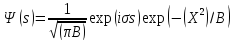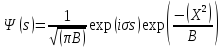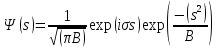, (1)

where s represents x or t for the spatial or temporal transforms, respectively, and represents angular frequency  or wavenumber k. B, the bandwidth parameter, was assigned a value of 4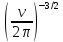for the temporal transform and 1.5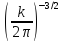for the temporal transform. Conclusions are not sensitive to these arbitrarily assigned values of B, but much larger values reduce the amplitude contrast in time of signals and enhance a ringing effect, and substantially smaller values do not sufficiently resolve large-scale or low frequency waves. The transform is obtained by taking the time-centered dot product of the wavelet and all daily consecutive overlapping time series segments at each grid point around the globe, then applying a similar transform in longitude to the result by the same approach. The transform for a selected wave number and frequency is calculated for every day and at every longitude grid point in the 7.5S to 7.5N averaged OLR anomaly data from 1975 to 2009. Averaging OLR over 7.5S to 7.5N increases the likelihood that the dominant coherent signals are Kelvin waves because this average acts as a filter for cross equatorial symmetry, and proximity to the equator reduces the net contribution of extratropical waves.

b. Linear Regression Models

Simple linear regression is frequently applied to diagnose structures that are coherent with filtered signals (e.g., Hendon and Salby 1994; Wheeler et al. 2000). In this analysis, the space-time wavelet transform at a selected longitude, wave number, and frequency, becomes a base index time series for regression models at each grid point over a range of longitudes and pressure levels. Either the real or imaginary parts of the transform work for this index. The imaginary part produces convenient zonal phasing in the regression maps, but the conclusions are the same regardless of this choice. Base longitudes are at each 2.5 grid point from 60E to 90E. This focus on the Indian basin reduces the contribution of extratropical features that are much more pronounced over the western hemisphere. Calculating regression models at each base point, then averaging over all of them reduces local disconformities, yielding conclusions less sensitive to geography. The time series from each of those points serve as predictors in regression models at each grid point across a broad geographical domain to diagnose the associated structures. One grid of regression models is calculated for each base point time series. To illustrate, the algorithm models the variable Y at the grid point S as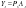, (2)

where Px is a matrix whose first column is a list of ones and second column is the base index at the longitude grid point x. As is a vector of regression coefficients at the grid point S. After solving for As at each grid point by matrix inversion, (2) is then applied as a scalar equation to diagnose wave structure by substituting a single value for the second column of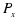that is representative of a crest of a wave located at the base longitude (its value is set here at +1 standard deviation). These regression models are applied to create ‘composite’ anomalies of OLR, u and v winds, and geopotential height. Results are calculated for the region 180 to the east and west of each base longitude, and then the set of results from all base points are averaged, following Roundy and MacRitchie (2012). The statistical significance of the difference from zero of the result at each point on the map is assessed based on the correlation coefficient (e.g., Wilks 2011), and I analyze and discuss only those regressed signals that are deemed to be significantly different from zero at the 90% level. This significance test is completed for each individual regression map before averaging over results from each base point, so that the number of degrees of freedom is not inflated by inclusion of the same wave events at multiple grid points. Since the regression is accomplished in the time domain, some signal from wave numbers other than the target wave number can appear in results if they tend to occur together in a particular pattern (Wheeler et al. 2000). Such regression results will be most reliable close to the centers of the composites because spreading will occur due to the episodic nature of convection and variations in the background state. Amplitudes of regressed anomalies following this approach are much smaller than those of other authors who have regressed signals against OLR data filtered for the broader Kelvin band of Wheeler and Kiladis (1999) because the full band includes much more variance than is retained at just one wave number and frequency.

The above approach diagnoses the spatial structures associated with signals along the dispersion curves of shallow water model Kelvin wave solutions at particular equivalent depths. For reference, we also calculate a composite MJO following a similar approach, by replacing the base index time series with MJO band pass filtered OLR anomalies averaged from 10N to 10S. The MJO band signal is averaged over a broader latitude band than are Kelvin signals in this work in order to capture the signals of some MJO events that have OLR anomalies shifted farther into one hemisphere. The MJO band is defined as wave numbers 0-9 eastward and periods of 30-100 days. The value of −1 standard deviation in the base index is then substituted to generate a map. Base points for the MJO composite are selected from 70E to 110E in order to reduce contamination of the western portion of the regression maps by Africa. Kelvin band signals at higher wave numbers did not require such an eastward shift because the wavelengths assessed for them yielded less contamination from Africa.

A variation on the above regression approach is also applied here to diagnose how signals along the Kelvin wave dispersion curves at particular equivalent depths vary with the phase of the MJO. An index of OLR anomaly data filtered for the wave number frequency band of the MJO at 80E is assigned as P in equation (2) and applied to predict Y, which is assigned to be the absolute value of the sum of the real and imaginary parts of the wavelet transform at a selected wave number and frequency at a time lag. Averaging regression maps over multiple base points is not applied for this analysis since the associated geographical signals is the target outcome. The value of −1 standard deviation is then substituted for the second column of P at each grid point and time lag. After discarding the time local mean of the result, it shows how the timing of changes in activity in Kelvin waves characterized by particular phase speeds varies with the MJO.

1   2   3   4   5